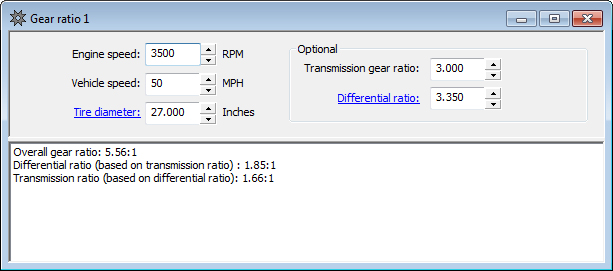## Gear Ratio Calculation

Observed speed gear ratio calculatorIn addition to calculating the overall gear ratio, this information can be used to calculate either the transmission gear ratio or the differential ratio. The differential ratio is calculated from the transmission gear ratio, and the transmission gear ratio is calculated from the differential gear ratio.

Enter your engine speed, vehicle speed, and tire diameter. Calculate your overall gear ratio. You can also enter your transmission gear ratio and differential ratio and calculate each one from the other.